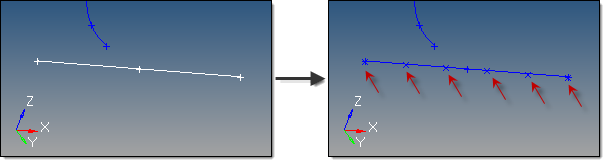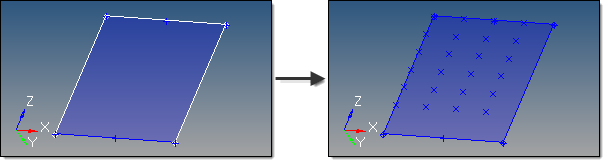﻿ extract parametric subpanel (Points panel)
Manufacturing Solutions

# extract parametric subpanel (Points panel)# extract parametric subpanel (Points panel)This subpanel creates free points at parametric locations on lines and surfaces.

For lines:

 • 0.0 <= u lower bound <= u upper bound <= 1.0.
 • If the lower and upper bounds are the same, only one node is created.
 • If the number of u nodes is specified as 1, only the lower bound is used.
 • The line parameterization type can be specified as either arc length or internal.This example shows u ranging from 0 to 1, with six u points, created evenly along the arc length.

For surfaces:

 • 0.0 <= u lower bound <= u upper bound <= 1.0.
 • 0.0 <= v lower bound <= v upper bound <= 1.0.
 • The number of u and v free points must be >= 1.
 • The total number of free points created equals (number of u points * number of v points).
 • The surface parametric area is scaled to the visible surface area. Free points will be created inside the visible surface area.This examples uses u from 0 to 0.75 with 4 u points, and v from 0.25 to 1.0 with 6 v points.